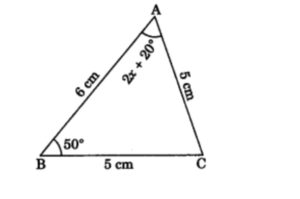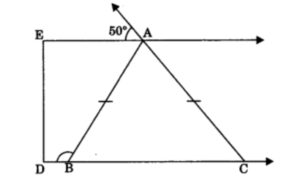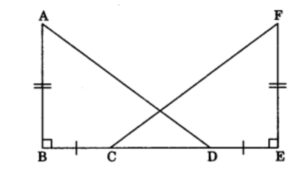## MCQ Questions of Class 9 Maths Chapter 7 Triangles with Answers

Free PDF Download of CBSE Class 9 Maths Chapter 7 Triangles Multiple Choice Questions with Answers.Based on Latest Exam Pattern. Students can solve NCERT Class 9 Maths Chapter 7 Triangles Multiple Choice Questions with Answers to know their preparation level.

# Triangles Class 9 MCQs Questions with Answers

MCQ Questions for Class 9 Maths Chapter 7 Triangles with Answers

1. In ∆ PQR, ∠R = ∠P and QR = 4 cm and PR = 5 cm. Then the length of PQ is

2. If ABC and DBC are two isosceles triangles on the same base BC. Then:

3. In given figure, the measure of ∠BAC is4. Two sides of a triangle are of lengths 5 cm and 1.5 cm. The length of the third side of the triangle cannot be

5. In figure X is a point in the interior of square ABCD, AXYZ is also a square. If DY = 3 cm and AZ = 2 cm, then find BY.6. A triangle in which two sides are equal is called:

7. In figure if AE || DC and AB = AC, find the value of ∠ABD.8. It is given that ΔABC = ΔFDE and AB = 5 cm, ∠B = 40° and ∠A = 80°. Then which of the following is true?9. In ΔABC, AB = AC and ∠B = 50°, then find ∠C.

10. In triangle ABC, if AB=BC and ∠B = 70°, ∠A will be:

11. In a right triangle, the longest side is:

12. In figure AB ⊥ AE, BC ⊥ AB, CE = DE and ∠AED = 120°, then find ∠ECD.13. The angles opposite to equal sides of a triangle are:

14. In ΔABC, ∠C = ∠A and BC = 4 cm and AC = 5 cm, then find length of AB.

15. In figure AB ⊥ BE and EF ⊥ BE. If BC = DE and AB = EF, then ΔABD is congruent to16. D is a point on the side BC of a ΔABC such that AD bisects ∠BAC. Then

17. For two triangles, if two angles and the included side of one triangle are equal to two angles and the included side of another triangle. Then the congruency rule is:

18. ABC is an isosceles triangle in which altitudes BE and CF are drawn to equal sides AC and AB, respectively. Then:

19. In triangles ABC and DEF, AB = FD and ∠A = ∠D. The two triangles will be congruent by SAS axiom if

20. In triangles ABC and PQR, AB = AC, ∠C = ∠P and ∠B = ∠Q. The two triangles are

Hope the information shed above regarding MCQ Questions for Class 9 Maths Chapter 7 Triangles with Answers Pdf free download has been useful to an extent. If you have any other queries of CBSE Class 9 Maths Chapter 7 Triangles MCQs Multiple Choice Questions with Answers, feel free to comment below so that we can revert back to us at the earliest possible

By Team Study Rate